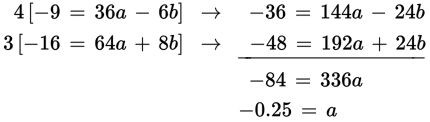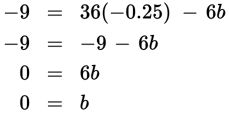# SAT Math Multiple Choice Question 566: Answer and Explanation

### Test Information

Question: 566

11. If the graph of the equation y = ax2 + bx + c passes through the points (0, 2), (–6, –7), and (8, –14), what is the value of a + b + c?

• A. –19
• B. –2
• C. 1.75
• D. 2.25

Explanation:

C

Difficulty: Hard

Strategic Advice: Writing quadratic equations can be tricky and time-consuming. If you know the roots, you can use factors to write the equation. If you don't know the roots, you need to create a system of equations to find the coefficients of the variable terms.

Getting to the Answer: You don't know the roots of this equation, so start with the point that has the nicest values (0, 2) and substitute them into the equation, y = ax 2 + bx + c, to get 2 = a(0)2 + b(0) + c, or 2 = c. Now your equation looks like y = ax 2 + bx + 2. Next, use the other two points to create a system of two equations in two variables.

(-6, -7) → -7 = a(-6)2 + b(-6) + 2 → -9 = 36a - 6b
(8, -14) → -14 = a(8)2 + b(8) + 2 → -16 = 64a + 8b

You now have a system of equations to solve. If you multiply the top equation by 4 and the bottom equation by 3, and then add the equations, the b terms will eliminate each other.Now, find b by substituting a = –0.25 into either of the original equations. Using the top equation, you get:The value of a + b + c is (–0.25) + 0 + 2 = 1.75.